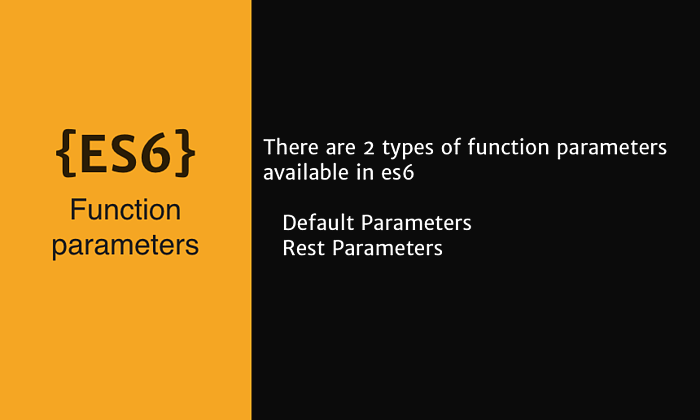# Function parameters in es6

Wed Jul 20 2016There are 2 types of function parameters available in es6,

• Default Parameters
• Rest Parameters

## Default Parameter

Default parameter are a way to pass a value to the function parameter when there is no value is being passed by the callee during invocation.

We specify this value when defining a function,

``````function printName(name = 'there') {
return `Hello,  \${name}`
}
``````

We can even use the default parameter of one parameter as part of an expression for another paramter. For eg:

``````function printName(name = 'there', fullname = name + ' lastName') {
return `Hello,  \${name} -  \${fullname} `
}
``````

## Rest Parameters

The rest parameter syntax allows us to represent an indefinite number of arguments as an array.

This is achieved by using a new operator called Spread Operator which is represented by `...` 3dots

Spread Operator It is used when we want to pass an array to a function as individual arguments of that function. For eg:

``````function sum(x, y, z) {
return x + y + z
}
let values = [1, 2, 3]
// notice the usage of ...
sum(...values)
``````

Another Example

``````function f(x, ...y) {
// y is an Array
return x * y.length
}
f(3, 'hello', true) == 6
``````

If the last named argument of a function is prefixed with `...`, it becomes an array whose elements from 0 (inclusive) to `theArgs.length` (exclusive) are supplied by the actual arguments passed to the function.

In the above example, `theArgs` would collect the third argument of the function (because the first one is mapped to a, and the second to b) and all the consecutive argument